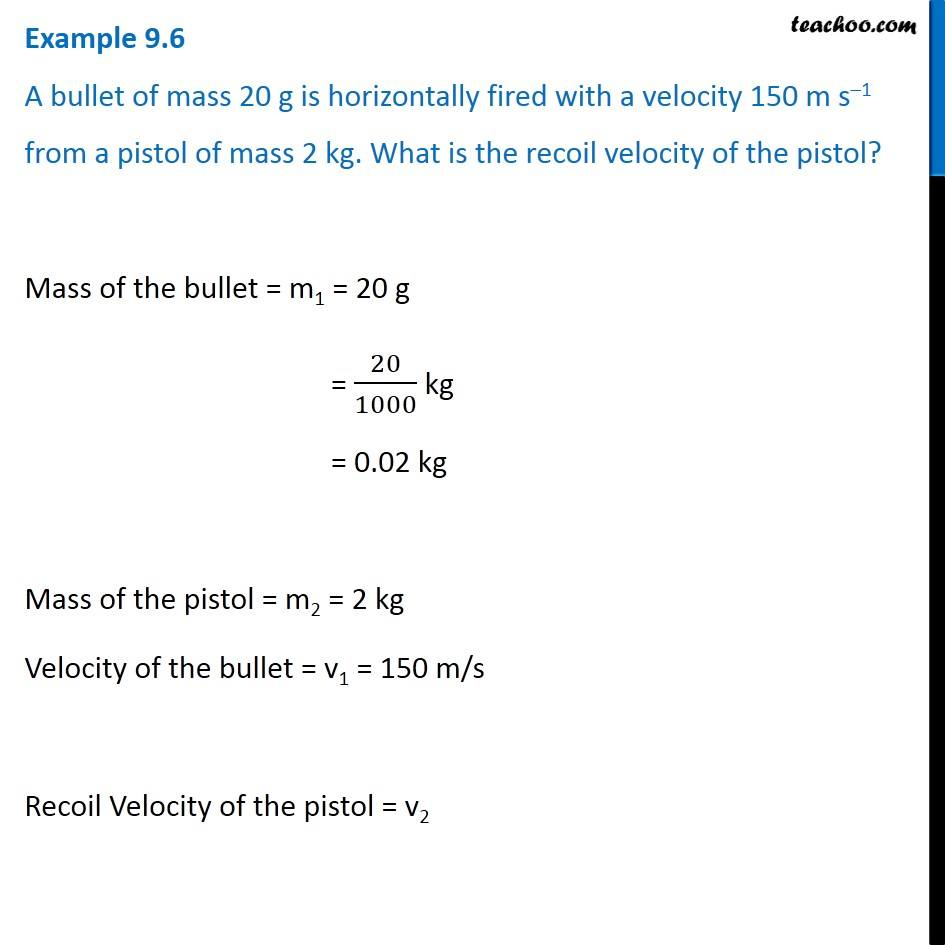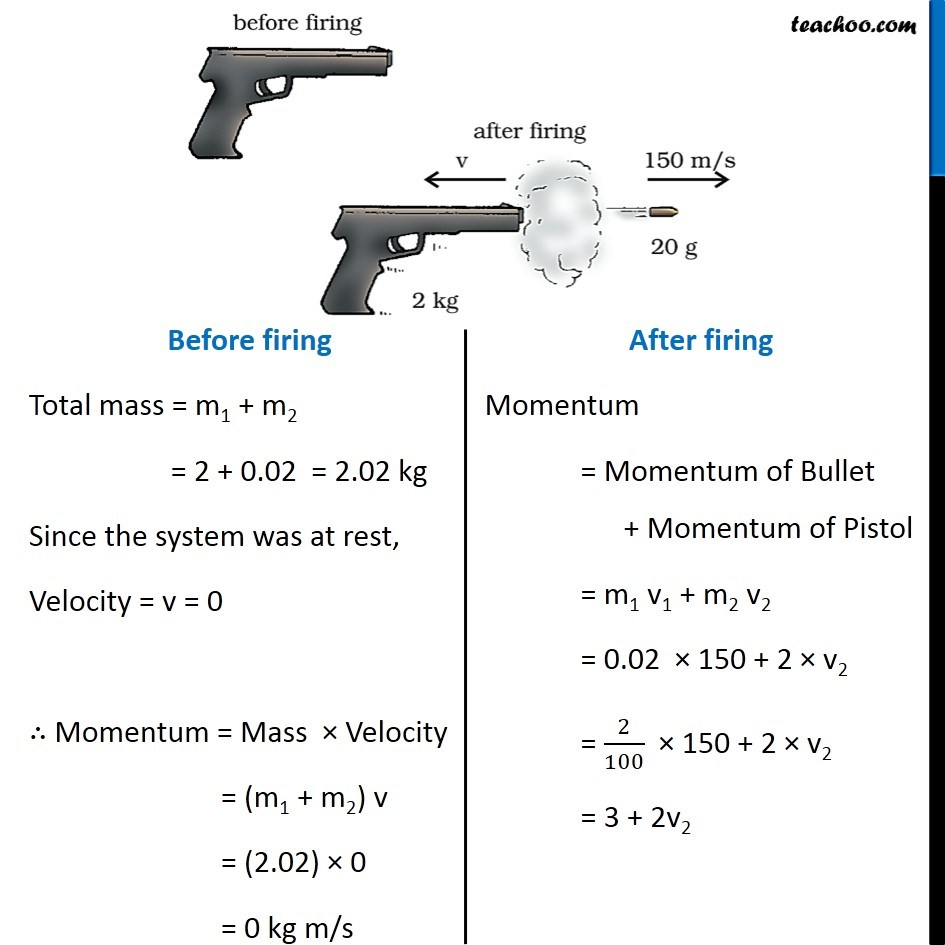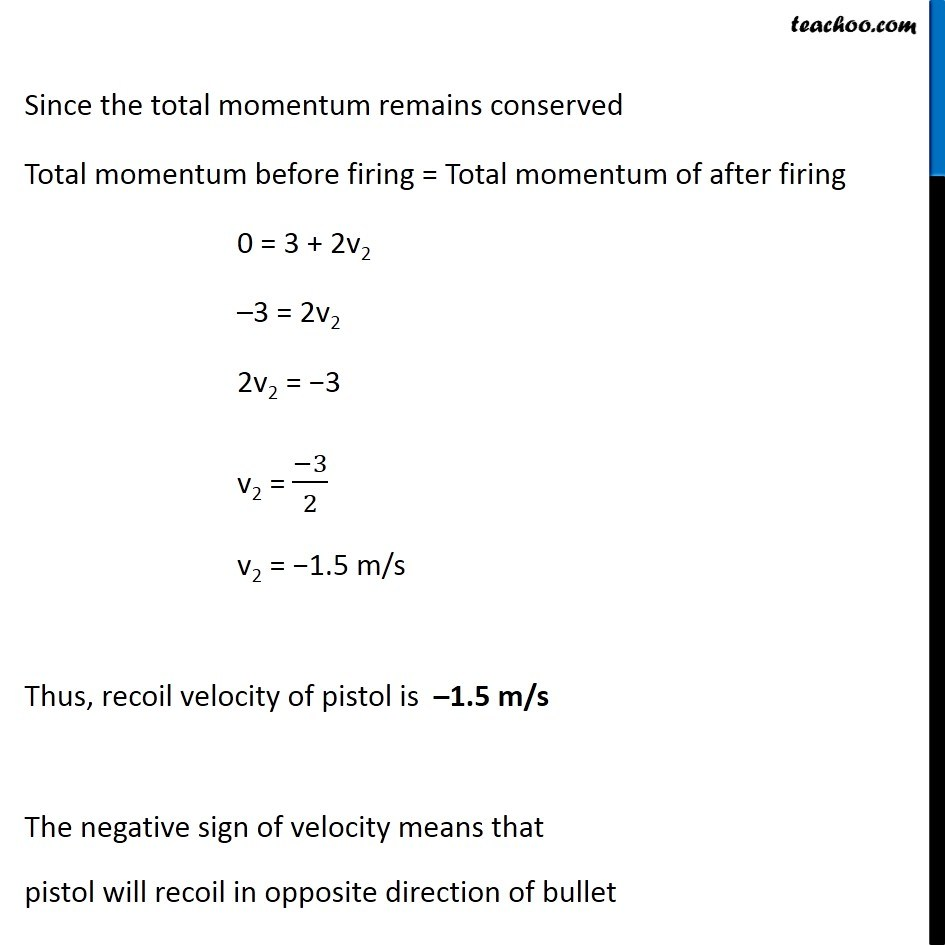Examples from NCERT Book

Class 9
Chapter 9 Class 9 - Force and Laws Of MotionLearn in your speed, with individual attention - Teachoo Maths 1-on-1 Class

### Transcript

Example 9.6 A bullet of mass 20 g is horizontally fired with a velocity 150 m s–1 from a pistol of mass 2 kg. What is the recoil velocity of the pistol? Mass of the bullet = m1 = 20 g = 20/1000 kg = 0.02 kg Mass of the pistol = m2 = 2 kg Velocity of the bullet = v1 = 150 m/s Recoil Velocity of the pistol = v2 Before firing Total mass = m1 + m2 = 2 + 0.02 = 2.02 kg Since the system was at rest, Velocity = v = 0 ∴ Momentum = Mass × Velocity = (m1 + m2) v = (2.02) × 0 = 0 kg m/s After firing Momentum = Momentum of Bullet + Momentum of Pistol = m1 v1 + m2 v2 = 0.02 × 150 + 2 × v2 = 2/100 × 150 + 2 × v2 = 3 + 2v2 Since the total momentum remains conserved Total momentum before firing = Total momentum of after firing 0 = 3 + 2v2 –3 = 2v2 2v2 = −3 v2 = (−3)/2 v2 = −1.5 m/s Thus, recoil velocity of pistol is –1.5 m/s The negative sign of velocity means that pistol will recoil in opposite direction of bullet